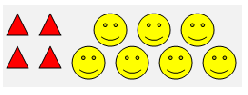# Ratio Review

1) Write the ratio in another form.  9:6

2)  Write the ratio of triangles to smiley faces.3)  There are 145 students in your school chorus program.  25 students sing soprano and 15 sing alto.  Write a ratio of the number of sopranos to all the students in the chorus program.  Write your answer in lowest terms.
4)  Make the ratios equal.  9/25 = 36/?
5)  The ratio of apples to oranges in a fruit bowl is 1 to 4.   If there are 5 apples in the bowl, how many oranges are there?
6)  2 pounds of apples cost \$0.76.  How much would 3.5 pounds cost?